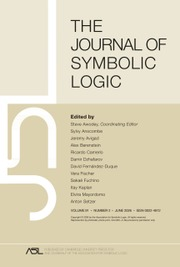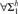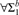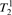Skip to main content Accessibility help
Home
Hostname: page-component-564cf476b6-jjt9s Total loading time: 2.366 Render date: 2021-06-19T01:40:36.349Z Has data issue: true Feature Flags: { "shouldUseShareProductTool": true, "shouldUseHypothesis": true, "isUnsiloEnabled": true, "metricsAbstractViews": false, "figures": true, "newCiteModal": false, "newCitedByModal": true, "newEcommerce": true }The Journal of Symbolic Logic

# FRAGMENTS OF APPROXIMATE COUNTING

Published online by Cambridge University Press:  25 June 2014

Corresponding
E-mail address:

## Abstract

We study the long-standing open problem of giving$\forall {\rm{\Sigma }}_1^b$ separations for fragments of bounded arithmetic in the relativized setting. Rather than considering the usual fragments defined by the amount of induction they allow, we study Jeřábek’s theories for approximate counting and their subtheories. We show that the$\forall {\rm{\Sigma }}_1^b$ Herbrandized ordering principle is unprovable in a fragment of bounded arithmetic that includes the injective weak pigeonhole principle for polynomial time functions, and also in a fragment that includes the surjective weak pigeonhole principle for FPNP functions. We further give new propositional translations, in terms of random resolution refutations, for the consequences of$T_2^1$ augmented with the surjective weak pigeonhole principle for polynomial time functions.

## Keywords

Type
Articles
Information
The Journal of Symbolic Logic , June 2014 , pp. 496 - 525
Copyright
Copyright © The Association for Symbolic Logic 2014

## Access options

Get access to the full version of this content by using one of the access options below.

## References

Beckmann, A. and Buss, S., Polynomial local search in the polynomial hierarchy and witnessing in fragments of bounded arithmetic, Journal of Mathematical Logic, vol. 9 (2009), pp. 103138.CrossRefGoogle Scholar
Beckmann, A. and Buss, S., Characterization of definable search problems in bounded arithmetic via proof notations, Ways of Proof Theory, Ontos Verlag, Frankfurt 2010, pp. 65134.Google Scholar
Ben-Sasson, E. and Wigderson, A., Short proofs are narrow—resolution made simple, Journal of the ACM, vol. 48 (2001), pp. 149169.CrossRefGoogle Scholar
Boughattas, S. and Kołodziejczyk, L. A., The strength of sharply bounded induction requires MSP, Annals of Pure and Applied Logic, vol. 161 (2010), pp. 504510.CrossRefGoogle Scholar
Buss, S., Bounded Arithmetic, Bibliopolis, Napoli 1986.Google Scholar
Buss, S., Axiomatizations and conservation results for fragments of bounded arithmetic, In Logic and Computation, Proceedings of a workshop held at Carnegie Mellon University, American Mathematical Society, 1990, pp. 5784.CrossRefGoogle Scholar
Buss, S., First-order proof theory of arithmetic, In Handbook of Proof Theory (Buss, S., editor), Elsevier, Amsterdam 1998, pp. 79147.Google Scholar
Buss, S. and Krajíček, J., An application of Boolean complexity to separation problems in bounded arithmetic, Proceedings of the London Mathematical Society, vol. 69 (1994), pp. 121.CrossRefGoogle Scholar
Chiari, M. and Krajíček, J., Witnessing functions in bounded arithmetic and search problems, this Journal, vol. 63 (1998), pp. 10951115.
Chiari, M. and Krajíček, J., Lifting independence results in bounded arithmetic, Archive for Mathematical Logic, vol. 38 (1999), pp. 123138.CrossRefGoogle Scholar
Hájek, P. and Pudlák, P., The Metamathematics of First Order Arithmetic, Springer, Berlin 1993.CrossRefGoogle Scholar
Jeřábek, E., Dual weak pigeonhole principle, Boolean complexity, and derandomization, Annals of Pure and Applied Logic, vol. 129 (2004), pp. 137.CrossRefGoogle Scholar
Jeřábek, E., The strength of sharply bounded induction, Mathematical Logic Quarterly, vol. 52 (2006), pp. 613624.CrossRefGoogle Scholar
Jeřábek, E., Approximate counting in bounded arithmetic, this Journal, vol. 72 (2007), pp. 959993.
Jeřábek, E., On independence of variants of the weak pigeonhole principle, Journal of Logic and Computation, vol. 17 (2007), pp. 587604.CrossRefGoogle Scholar
Jeřábek, E., Approximate counting by hashing in bounded arithmetic, this Journal, vol. 74 (2009), pp. 829860.
Kołodziejczyk, L. A., Nguyen, P., and Thapen, N., The provably total NP search problems of weak second-order bounded arithmetic, Annals of Pure and Applied Logic, vol. 162 (2011), pp. 419446.CrossRefGoogle Scholar
Krajíček, J., No counter-example interpretation and interactive computation, In Logic from Computer Science (Moschovakis, Y., editor), vol. 21 (1992), Mathematical Sciences Research Institute Publications, Springer, Berlin pp. 287293.Google Scholar
Krajíček, J., Lower bounds to the size of constant-depth propositional proofs, this Journal, vol. 59 (1994), pp. 7386.
Krajíček, J., Bounded Arithmetic, Propositional Logic and Computational Complexity, Cambridge University Press, 1995.Google Scholar
Krajíček, J., On the weak pigeonhole principle, Fundamenta Mathematicae, vol. 170 (2001),pp. 123140.CrossRefGoogle Scholar
Krajíček, J., Pudlák, P., and Takeuti, G., Bounded arithmetic and the polynomial hierarchy, Annals of Pure and Applied Logic, vol. 52 (1991), pp. 143153.CrossRefGoogle Scholar
Lauria, M., Short Res*(polylog) refutations if and only if narrow Res refutations, 2011, available at arXiv:1310.5714.
Maciel, A., Pitassi, T., and Woods, A., A new proof of the weak pigeonhole principle, Proceedings of the 32nd Annual ACM Symposium on Theory of Computing, 2000, pp. 368377.
Parikh, R., Existence and feasibility in arithmetic, this Journal, vol. 36 (1971), pp. 494508.
Paris, J., Wilkie, A., and Woods, A., Provability of the pigeonhole principle and the existence of infinitely many primes, this Journal, vol. 53 (1988), pp. 12351244.
Pudlák, P., Ramsey’s theorem in bounded arithmetic, In Computer Science Logic: Proceedings of the 4th Workshop, CSL ’90 (Börger, E., Büning, H. Kleine, Richter, M., and Schönfeld, W., editors), Springer, Berlin 1991, pp. 308317.Google Scholar
Pudlák, P., Some relations between subsystems of arithmetic and the complexity of computations, Logic From Computer Science: Proceedings of a Workshop held November 13-17, 1989, Mathematical Sciences Research Institute Publication #21, Springer-Verlag, Berlin 1992, pp. 499519.Google Scholar
Pudlák, P., Consistency and games—in search of new combinatorial principles, In Logic Colloquium ’03 (Stoltenberg-Hansen, V. and Väänänen, J., editors), Lecture Notes in Logic, no. 24, Association of Symbolic Logic, 2006, pp. 244281.Google Scholar
Pudlák, P. and Thapen, N., Alternating minima and maxima, Nash equilibria and bounded arithmetic, Annals of Pure and Applied Logic, vol. 163 (2012), pp. 604614.CrossRefGoogle Scholar
Riis, S., Making infinite structures finite in models of second order bounded arithmetic, In Arithmetic, Proof Theory, and Computational Complexity (Clote, P. and Krajíček, J., editors), Oxford University Press, Oxford 1993, pp. 289319.Google Scholar
Segerlind, N., Buss, S., and Impagliazzo, R., A switching lemma for small restrictions and lower bounds for k-DNF resolution, SIAM Journal on Computing, vol. 33 (2004), pp. 11711200.CrossRefGoogle Scholar
Skelley, A. and Thapen, N., The provably total search problems of bounded arithmetic, Proceedings of the London Mathematical Society, vol. 103 (2011), pp. 106138.CrossRefGoogle Scholar
Thapen, N., A model-theoretic characterization of the weak pigeonhole principle, Annals of Pure and Applied Logic, vol. 118 (2002), pp. 175195.CrossRefGoogle Scholar
Thapen, N., Structures interpretable in models of bounded arithmetic, Annals of Pure and Applied Logic, vol. 136 (2005), pp. 247266.CrossRefGoogle Scholar
8
Cited by

# Send article to Kindle

To send this article to your Kindle, first ensure no-reply@cambridge.org is added to your Approved Personal Document E-mail List under your Personal Document Settings on the Manage Your Content and Devices page of your Amazon account. Then enter the ‘name’ part of your Kindle email address below. Find out more about sending to your Kindle. Find out more about sending to your Kindle.

Note you can select to send to either the @free.kindle.com or @kindle.com variations. ‘@free.kindle.com’ emails are free but can only be sent to your device when it is connected to wi-fi. ‘@kindle.com’ emails can be delivered even when you are not connected to wi-fi, but note that service fees apply.

Find out more about the Kindle Personal Document Service.

FRAGMENTS OF APPROXIMATE COUNTING
Available formats
×

# Send article to Dropbox

To send this article to your Dropbox account, please select one or more formats and confirm that you agree to abide by our usage policies. If this is the first time you use this feature, you will be asked to authorise Cambridge Core to connect with your <service> account. Find out more about sending content to Dropbox.

FRAGMENTS OF APPROXIMATE COUNTING
Available formats
×

# Send article to Google Drive

To send this article to your Google Drive account, please select one or more formats and confirm that you agree to abide by our usage policies. If this is the first time you use this feature, you will be asked to authorise Cambridge Core to connect with your <service> account. Find out more about sending content to Google Drive.

FRAGMENTS OF APPROXIMATE COUNTING
Available formats
×
×

#### Reply to:Submit a response

Please enter your response.

#### Your details

Please enter a valid email address.

#### Conflicting interests

Do you have any conflicting interests? *